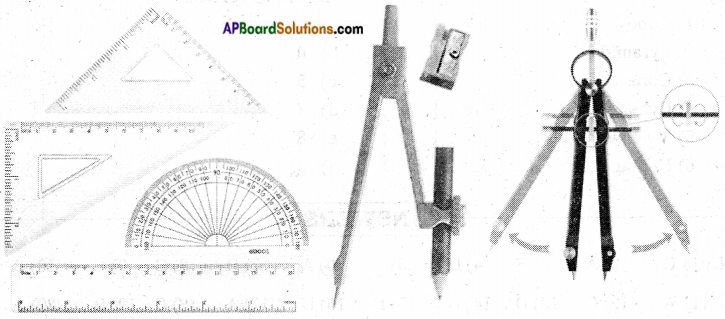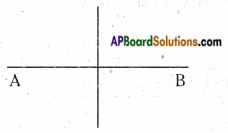# AP Board 6th Class Maths Notes Chapter 10 Practical Geometry

Students can go through AP Board 6th Class Maths Notes Chapter 10 Practical Geometry to understand and remember the concepts easily.

## AP State Board Syllabus 6th Class Maths Notes Chapter 10 Practical Geometry→ The above are the components we see in a geometry box. They are pair of set squares, protractor, graduated ruler, compasses and the divider.

→ Graduated ruler: A scale is used to draw straight edges of given length. It is also used to measure the lengths of the given line segments. A scale is also called a graduated ruler.

→ Compass: A compass is used to draw a circle of given radius. Sometimes we draw only a part of a circle which is called an arc. A compass is used in the construction of a line segment of given length, a perpendicular to a given line, given angle and in many more geometrical shapes.→ Divider: A divider is used to measure the lengths of straight line segments and curved lines. It is also used to compare the lengths of two line segments.

→ Set squares: The pair of set squares is used to draw the pair of parallel lines.

→ Perpendicular bisector: The perpendicular bisector of a given line segment is the line which divides the given line segment into two equal parts at right angles.→ Angle bisector: Angle bisector is a ray which divides the given angle into two equal parts.